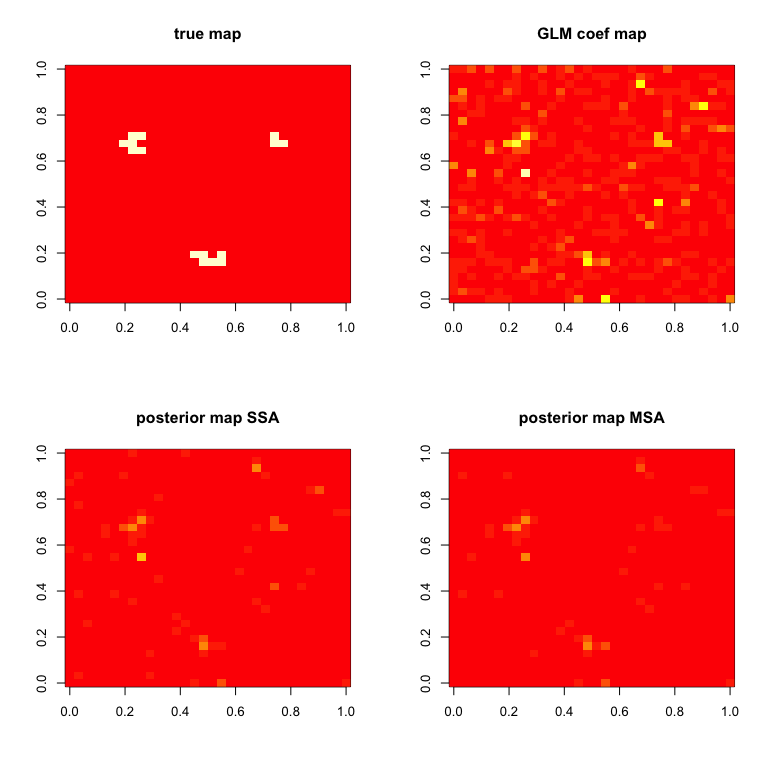BHMSMAfMRI performs Bayesian hierarchical multi-subject multiscale analysis of function MRI (fMRI) data as described in Sanyal & Ferreira (2012), or other multiscale data, using wavelet based prior that borrows strength across subjects and provides posterior smooth estimates of the effect sizes and samples from their posterior distribution.

## Installation

### Install from CRAN

install.packages("BHMSMAfMRI")

### Install from GitHub

# install.packages("devtools")
devtools::install_github("nilotpalsanyal/BHMSMAfMRI")

## The main function:

BHMSMA is the main function which accepts fMRI data as a 4D array (see code below) and a design matrix. For the time-series of all voxels, a general linear model (GLM) is fit with all the regressors in the design matrix. After that, the standardized regression coefficient map of a regressor of interest is subjected to further analysis. The function BHMSMA returns the posterior smoothed map of the regression coefficients. Below is a basic illustration of its use. For a detailed manual, see the package vignette.

library(BHMSMAfMRI)
#>
#>  Welcome! Thanks for trying BHMSMAfMRI.
#>
#>  Website: https://nilotpalsanyal.github.io/BHMSMAfMRI/
#>  Bug report: https://github.com/nilotpalsanyal/BHMSMAfMRI/issues

# Read data from image files
fpath <- system.file("extdata", package="BHMSMAfMRI")
untar(paste0(fpath,"/fmridata.tar"), exdir=tempdir())
n <- 3
grid <- 32
ntime <- 9
data <- array(dim=c(n,grid,grid,ntime))
for(subject in 1:n)
{
directory <- paste0(tempdir(),"/fmridata","/s0",subject,"/")
nimages=9, dim.image=c(grid,grid,1))
data[subject,,,] <- a[,,1,]
}
data(fmridata)
names(fmridata)
#>  "grid"         "nsubject"     "TrueCoeff"    "DesignMatrix"
truecoef <- fmridata$TrueCoeff designmat <- fmridata$DesignMatrix

# Perform analyses
k <- 2  #consider the second regressor
analysis <- "multi"     #perform multi-subject analysis (MSA)
BHMSMAmulti <- BHMSMA(n, grid, data, designmat, k, analysis, truecoef)
analysis <- "single"     #perform single subject analysis (SSA)
BHMSMAsingle <- BHMSMA(n, grid, data, designmat, k, analysis, truecoef)

# Compare results for the first subject
zlim = c(0,max(abs(BHMSMAmulti$GLMCoefStandardized[1,,,k]))) par(mfrow=c(2,2)) image( truecoef[1,,],col=heat.colors(12),main="true map") image( abs(BHMSMAsingle$GLMCoefStandardized[1,,,k]),
col=heat.colors(8),zlim=zlim,main="GLM coef map")
image( abs(BHMSMAsingle$GLMcoefposterior[1,,]), col=heat.colors(8),zlim=zlim,main="posterior map SSA") image( abs(BHMSMAmulti$GLMcoefposterior[1,,]),
col=heat.colors(8),zlim=zlim,main="posterior map MSA")Sanyal, Nilotpal, and Ferreira, Marco A.R. (2012). Bayesian hierarchical multi-subject multiscale analysis of functional MRI data. Neuroimage, 63, 3, 1519-1531. https://doi.org/10.1016/j.neuroimage.2012.08.041